# Evaluate Fractional Indices

In this worksheet, students will learn to evaluate integers and fractions with a fractional index number.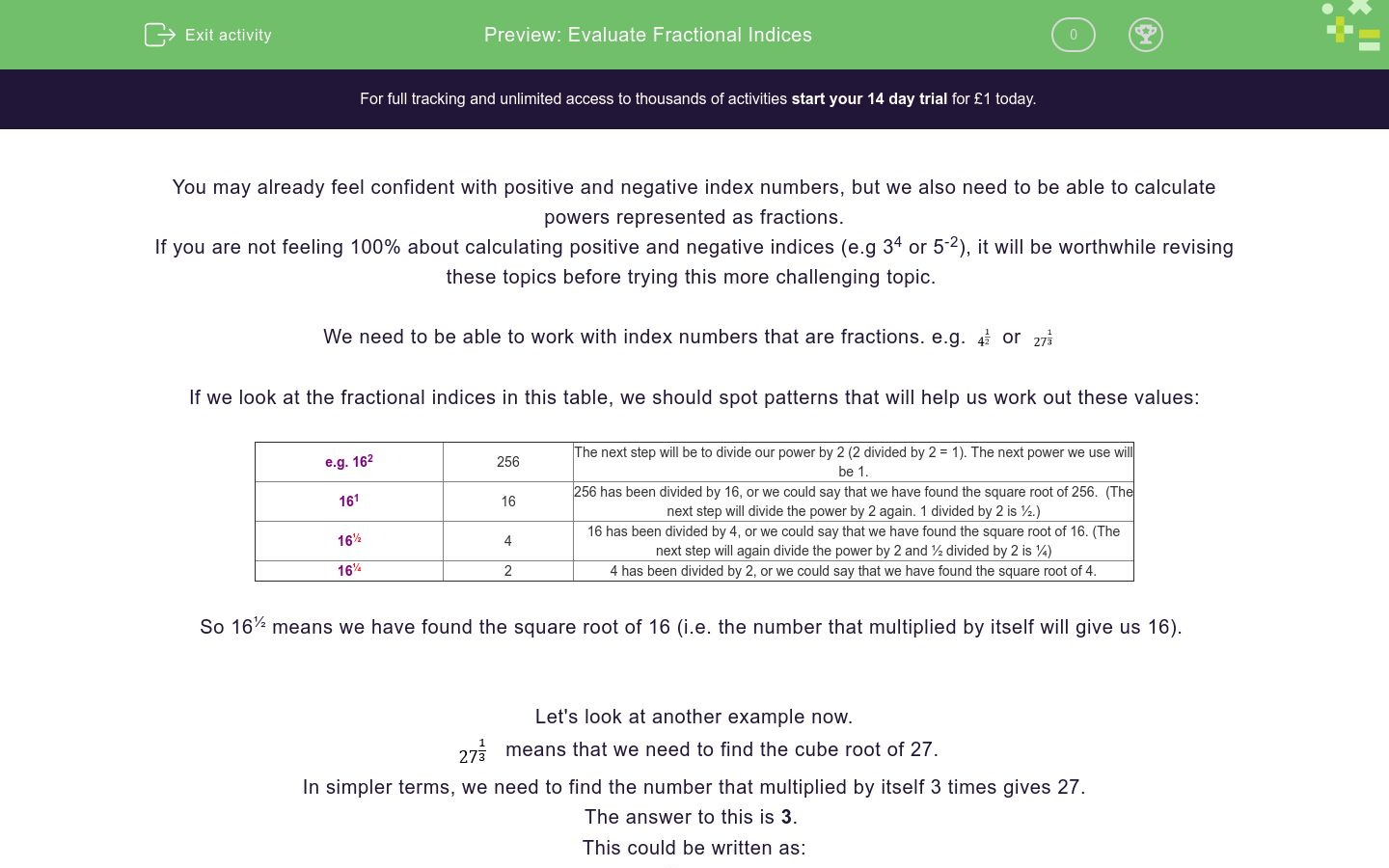Key stage:  KS 4

GCSE Subjects:   Maths

GCSE Boards:   Pearson Edexcel, OCR, Eduqas, AQA

Curriculum topic:   Number, Indices and Surds

Curriculum subtopic:   Structure and Calculation, Powers and Roots

Difficulty level:### QUESTION 1 of 10

You may already feel confident with positive and negative index numbers, but we also need to be able to calculate powers represented as fractions.

If you are not feeling 100% about calculating positive and negative indices (e.g 34 or 5-2), it will be worthwhile revising these topics before trying this more challenging topic.

We need to be able to work with index numbers that are fractions. e.g.orIf we look at the fractional indices in this table, we should spot patterns that will help us work out these values:

 e.g. 162 256 The next step will be to divide our power by 2 (2 divided by 2 = 1). The next power we use will be 1. 161 16 256 has been divided by 16, or we could say that we have found the square root of 256.  (The next step will divide the power by 2 again. 1 divided by 2 is ½.) 16½ 4 16 has been divided by 4, or we could say that we have found the square root of 16. (The next step will again divide the power by 2 and ½ divided by 2 is ¼) 16¼ 2 4 has been divided by 2, or we could say that we have found the square root of 4.

So 16½ means we have found the square root of 16 (i.e. the number that multiplied by itself will give us 16).

Let's look at another example now.means that we need to find the cube root of 27.

In simpler terms, we need to find the number that multiplied by itself 3 times gives 27.

The answer to this is 3

This could be written as:Here's one more example to check you've really got the hang of this.Let's try some questions now to put this into practise!

You may want to have a pen and paper so that you can compare your working to our explanations written by a teacher.

Imagine your friend has been working on evaluating:They are not sure how they should work this out, so they have 4 approaches listed below.

Which of these approaches is correct and has ensured they reached the correct answer?

24½ (because 49 x ½ = 24½)

49½ (because 49 + ½ = 49½ )

7 (because the square root of 49 is 7)

98 (because 49 divided by ½ = 98)

Evaluate:Hint: Start by finding:Let's move on now to consider fractional indices where the numerator isn't 1 now.

e.g.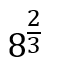There is an index law which will help us work out the value of this in steps: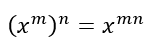When we have a numerator which is greater than 1, we can calculate as if it is 1 and then compensate afterwards.

So=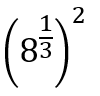becauseWe can now work out the cube root of 8 and then square it:Now apply this process, to work out the value of:Imagine your friend has been working on evaluating:They are not sure how they should work this out, so they have 3 approaches listed below.

Which of these approaches is correct and has ensured they reached the correct answer?

12.8 (because 32 x 2/5 = 12.8)

40.96 (because 32/5 squared = 40.96)

4 (because the 5th root of 32 is 2, and 2 squared is 4)

Complete the expression below:Remember to rewrite the power as a multiple of an easier fraction.

We can also be asked to find fractional powers of fractions!

e.g.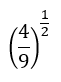In these cases we are being asked to find the square root of the fraction.

To find the root of a fraction, split the top (numerator) and bottom (denominator) into roots themselves.

Then calculate them separately and put them back together!

So our working would look like this:Here is another example to check you have it.

e.g. Evaluate:Choose the correct numerator and denominator in the table below.

 2 4 3 5 Numerator Denominator

We can use the same process when the fractional power has a numerator greater than 1.

e.g.We will write the original index number in a bracket like this:So what is the cube root of 8? (2)

And what is the cube root of 27? (3)Now for your chance to put this into practise by evaluating:Choose the correct numerator and denominator in the table below.

 5 25 9 3 Numerator Denominator

Now we need to combine what we have learnt about negative and fractional indices.

e.g. Evaluate:Using our index laws we could write this as: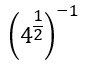We now need to find the square root of 4 and then find the reciprocal of this answer.

This is the working shown step by step:Choose the correct numerator and denominator in the table below.

 1 2 4 7 Numerator Denominator

We are now going to find a fraction raised to a negative fractional power.

e.g.To work this out, we need to find the square root of the numerator (√4 = 2) and the denominator (√9 = 3).

We should then find the reciprocal of 2/3, which is 3/2.

Let's see this process step-by-step:Choose the correct numerator and denominator in the table below.

 2 3 5 7 Numerator Denominator

And finally, we need to be able to work out the value of a fraction with a more complex negative fractional index number.

e.g.Firstly, find the cube roots of both the numerator and denominator separately.

3 x 3 x 3 = 27 so the cube root of 27 is 3.

5 x 5 x 5 = 125 so the cube root of 125 is 5.

Then we need to find the reciprocal, as we are working with a negative.

Finally, we find the square of this fraction.

Let's see this process shown step-by-step:Evaluate:Choose the correct numerator and denominator in the table below.

 9 3 36 6 Numerator Denominator
• Question 1

Imagine your friend has been working on evaluating:They are not sure how they should work this out, so they have 4 approaches listed below.

Which of these approaches is correct and has ensured they reached the correct answer?

7 (because the square root of 49 is 7)
EDDIE SAYS
We have been asked to work out 7½. Remember that the 'power of 1/2' means the same as 'find the square root'. This is the correct working which your friend should have:• Question 2

Evaluate:Hint: Start by finding:3
EDDIE SAYS
The 4th root of 81 means the same as 'find the number that multiplies by itself 4 times to give 81'. Does anything come to mind? The correct answer is 3 because: 3 x 3 x 3 x 3 = 81 Therefore, the 4th root of 81 = 3.
• Question 3

Let's move on now to consider fractional indices where the numerator isn't 1 now.

e.g.There is an index law which will help us work out the value of this in steps:When we have a numerator which is greater than 1, we can calculate as if it is 1 and then compensate afterwards.

So=becauseWe can now work out the cube root of 8 and then square it:Now apply this process, to work out the value of:9
EDDIE SAYS
Remember, work out the power as if it is a simple cube root, and then compensate afterwards. This is the correct working: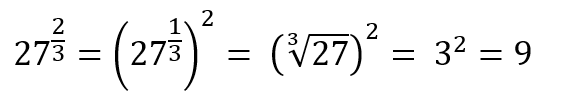• Question 4

Imagine your friend has been working on evaluating:They are not sure how they should work this out, so they have 3 approaches listed below.

Which of these approaches is correct and has ensured they reached the correct answer?

4 (because the 5th root of 32 is 2, and 2 squared is 4)
EDDIE SAYS
Option c was correct, as the answer is 4. This is the correct working which your friend should have:• Question 5

Complete the expression below:Remember to rewrite the power as a multiple of an easier fraction.

27
EDDIE SAYS
Did you spot that an 'easier fraction' to work with was 1/4? If you find the 4th root first, then you can cube your answer (times it by itself three times) to reach the solution. Your working should look something like this:• Question 6

We can also be asked to find fractional powers of fractions!

e.g.In these cases we are being asked to find the square root of the fraction.

To find the root of a fraction, split the top (numerator) and bottom (denominator) into roots themselves.

Then calculate them separately and put them back together!

So our working would look like this:Here is another example to check you have it.

e.g. Evaluate:Choose the correct numerator and denominator in the table below.

 2 4 3 5 Numerator Denominator
EDDIE SAYS
Remember that when we are finding a 4th root, we need to consider which number can be multiplied by itself four times to reach the number we are working with. In this case: 2 x 2 x 2 x 2 = 16 3 x 3 x 3 x 3 = 81 Here is an example of what your working should look close to:• Question 7

We can use the same process when the fractional power has a numerator greater than 1.

e.g.We will write the original index number in a bracket like this:So what is the cube root of 8? (2)

And what is the cube root of 27? (3)Now for your chance to put this into practise by evaluating:Choose the correct numerator and denominator in the table below.

 5 25 9 3 Numerator Denominator
EDDIE SAYS
Ask yourself: "What is the cube root of 27?" or "What can be multiplied by itself three times to reach 27?" Then do the same for 125. Your calculation should look something like this:• Question 8

Now we need to combine what we have learnt about negative and fractional indices.

e.g. Evaluate:Using our index laws we could write this as:We now need to find the square root of 4 and then find the reciprocal of this answer.

This is the working shown step by step:Choose the correct numerator and denominator in the table below.

 1 2 4 7 Numerator Denominator
EDDIE SAYS
Your process should look something like this: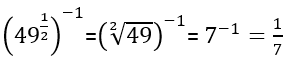• Question 9

We are now going to find a fraction raised to a negative fractional power.

e.g.To work this out, we need to find the square root of the numerator (√4 = 2) and the denominator (√9 = 3).

We should then find the reciprocal of 2/3, which is 3/2.

Let's see this process step-by-step:Choose the correct numerator and denominator in the table below.

 2 3 5 7 Numerator Denominator
EDDIE SAYS
Ask yourself: "What is the cube root of 27?" or "Which number can be multiplied by itself 3 times to reach 27?" (3) Then ask yourself the same question for 125 (5). Does your working look a bit like this?• Question 10

And finally, we need to be able to work out the value of a fraction with a more complex negative fractional index number.

e.g.Firstly, find the cube roots of both the numerator and denominator separately.

3 x 3 x 3 = 27 so the cube root of 27 is 3.

5 x 5 x 5 = 125 so the cube root of 125 is 5.

Then we need to find the reciprocal, as we are working with a negative.

Finally, we find the square of this fraction.

Let's see this process shown step-by-step:Evaluate:Choose the correct numerator and denominator in the table below.

 9 3 36 6 Numerator Denominator
EDDIE SAYS
Amazing work on this activity as these are not easy concepts! Don't be afraid to review the Level 1 or 2 activities if you found this tricky. Did your working look like this?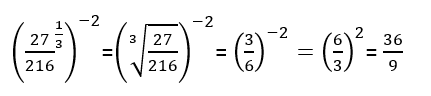---- OR ----

Sign up for a £1 trial so you can track and measure your child's progress on this activity.

### What is EdPlace?

We're your National Curriculum aligned online education content provider helping each child succeed in English, maths and science from year 1 to GCSE. With an EdPlace account you’ll be able to track and measure progress, helping each child achieve their best. We build confidence and attainment by personalising each child’s learning at a level that suits them.

Get started